# Colleges with the lowest SAT scores in Connecticut

Top 10 colleges in Connecticut with the lowest SAT scores
Looking for the colleges with the lowest SAT scores in Connecticut? Well you're in luck! We've compiled a national college database and have created a list of the top 10 universities with the lowest SAT scores in Connecticut below. If you are not a good test taker or worried about your test scores, this list is for you. These are the schools whose applicants had the lowest average SAT scores in Connecticut, which means that you can get into these colleges with a lower SAT score. We also include each college's ACT scores and acceptance rate so that you can see where you would have the easiest time getting in. Read on to find out more.

## Albertus Magnus College SAT scores

The average SAT score for Albertus Magnus College is 940.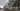The average SAT score of 940 breaks down into:

• SAT math: 460

The average ACT score for Albertus Magnus College is 24 and their acceptance rate is 58.1%.

## Southern Connecticut State University SAT scores

The average SAT score for Southern Connecticut State University is 1030.The average SAT score of 1030 breaks down into:

• SAT math: 500

The average ACT score for Southern Connecticut State University is 20 and their acceptance rate is 63.5%.

## University of Saint Joseph Connecticut SAT scores

The average SAT score for University of Saint Joseph Connecticut is 1070.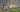The average SAT score of 1070 breaks down into:

• SAT math: 520

The average ACT score for University of Saint Joseph Connecticut is 21 and their acceptance rate is 90.3%.

## University of Hartford SAT scores

The average SAT score for University of Hartford is 1078.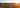The average SAT score of 1078 breaks down into:

• SAT math: 560

The average ACT score for University of Hartford is 24 and their acceptance rate is 80.9%.

## Western Connecticut State University SAT scores

The average SAT score for Western Connecticut State University is 1114.The average SAT score of 1114 breaks down into:

• SAT math: 550

The average ACT score for Western Connecticut State University is 22 and their acceptance rate is 79.2%.

## University of New Haven SAT scores

The average SAT score for University of New Haven is 1131.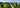The average SAT score of 1131 breaks down into:

• SAT math: 560

The average ACT score for University of New Haven is 23 and their acceptance rate is 83.9%.

## Quinnipiac University SAT scores

The average SAT score for Quinnipiac University is 1135.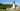The average SAT score of 1135 breaks down into:

• SAT math: 560

The average ACT score for Quinnipiac University is 24 and their acceptance rate is 78.5%.

## Eastern Connecticut State University SAT scores

The average SAT score for Eastern Connecticut State University is 1140.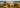The average SAT score of 1140 breaks down into:

• SAT math: 560

The average ACT score for Eastern Connecticut State University is 23 and their acceptance rate is 63.6%.

## Sacred Heart University SAT scores

The average SAT score for Sacred Heart University is 1175.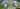The average SAT score of 1175 breaks down into:

• SAT math: 590

The average ACT score for Sacred Heart University is 25 and their acceptance rate is 60.5%.

## Fairfield University SAT scores

The average SAT score for Fairfield University is 1270.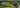The average SAT score of 1270 breaks down into:

• SAT math: 640